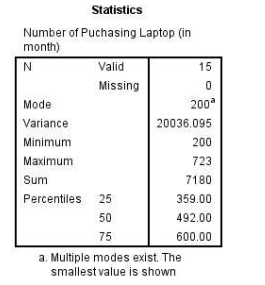Aug 23, 2023

QUESTION 6

There is an increasing trend in purchasing the laptop due to the pandemic Covid19. Hence, a group of researchers intends to describe this scenario by using descriptive statistics. SPSS output illustrating the information on the number of purchasing laptops (in a month) according to fifteen states in Malaysia is shown in the following output. Assume that the number of laptop purchases (in a month) is normally distributed.a) Calculate the mean and standard deviation for this study.

b) State the median value in this study. Hence interpret the value.

c) Construct a box and whisker plot for the above study.

d) Based on the plot in c), describe the shape of the distribution.

e) If the researchers wish to describe the shape of distribution by using an appropriate measurement, which descriptive measure would you suggest to the researchers?